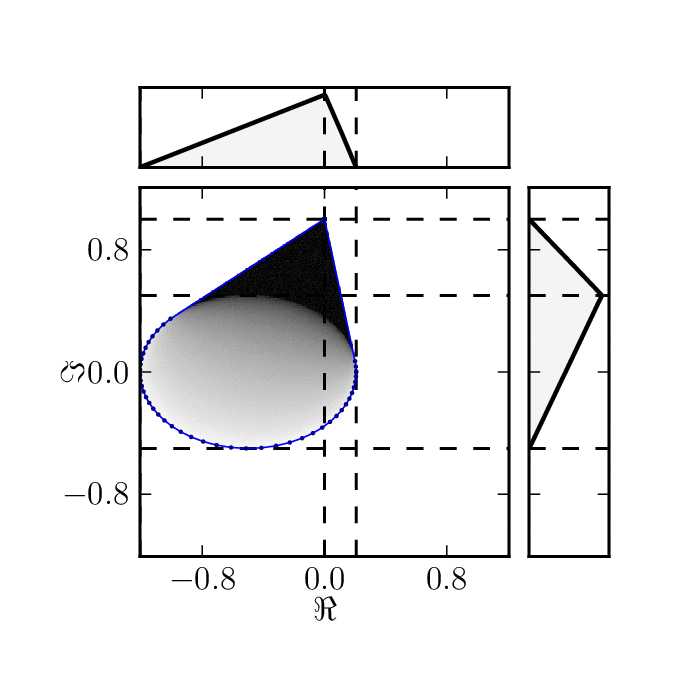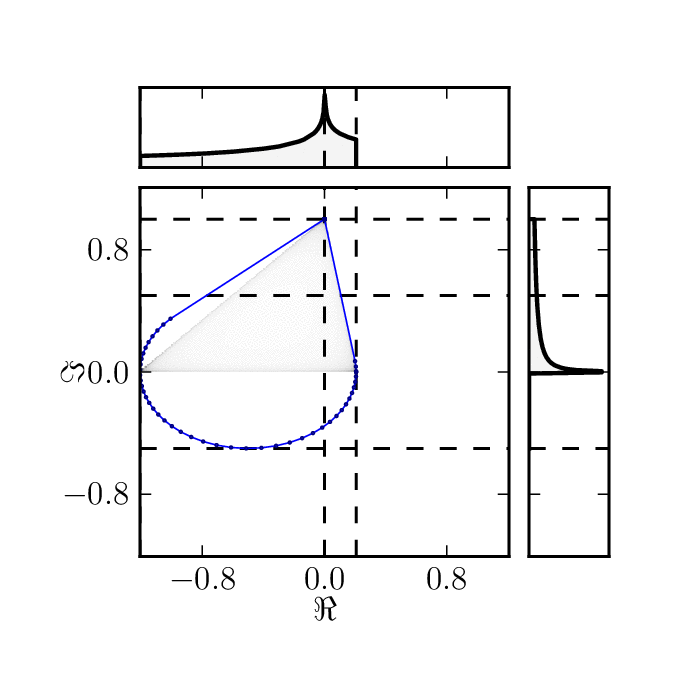Shadows of family of matrices $M_3(\phi)=e^{i\phi} \begin{pmatrix} -1&0&0\ 1&0&0\ 0&0&i \end{pmatrix},$ with $\phi\in[0, 2\pi]$. Upper and right plots present marginal distributions of real and imaginary part corresponding to shadows of hermitian and anti-hermitian parts of $M_3(\phi)$.

Standard numerical shadow with respect to complex states.Standard numerical shadow with respect to real states.